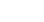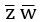October 19, 2023
October 19, 2023
October 19, 2023
###### Boolean-Algebra
October 19, 2023
 Question 8

The simultaneous equations on the Boolean variables x, y, z and w,

```      x + y + z = 1
xy = 0
xz + w = 1
xy += 0```

have the following solution for x, y, z and w, respectively.

 A 0 1 0 0 B 1 1 0 1 C 1 0 1 1 D 1 0 0 0
Question 8 Explanation:
Just put the values of each options in the equation and check it.
Question 8 Explanation:
Just put the values of each options in the equation and check it.